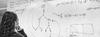# 1st Semester 2016/17: Computational Complexity Analysis of Logic Problems

Instructors
Ronald de Haan
ECTS
6
Description
Logic and computational complexity theory are closely related. On the one hand, many fundamental results in computational complexity theory make heavy use of various (fragments of) logics. For example, the development of the theory of NP-completeness is intimately linked to the satisfiability problem of propositional logic. On the other hand, when investigating different logics (especially those aimed at applications in a computer science setting), an important aspect to take into account is the computational complexity of various problems associated with these logics (e.g., satisfiability, model checking, entailment).

This project will allow students to learn how to analyze the computational complexity of (logic) problems in a hands-on manner. We will start with an introduction into the basic concepts of complexity theory, most notably polynomial-time reductions and NP-completeness. The students will each present an NP-completeness proof from the literature, allowing them to become more familiar with the general structure of such proofs. Further lectures cover several widely-used notions in complexity theory that go beyond NP-completeness (e.g., fixed-parameter tractability). These lectures build up to the final assignment of the project, where students perform a computational complexity analysis of a (logic) problem using the concepts learned in the course.

This project complements the Computational Complexity course in that it focuses on applying several main concepts in the area of computational complexity (that are dealt with theoretically in the Computational Complexity course) to concrete problems from different areas of logic research. The project is open both to students that have followed the Computational Complexity course and students that have not.

Prerequisites
There are no specific prerequisites.
Assessment
Students present one proof from the literature (20%), and write a final report where they describe how they analyzed the complexity of a problem of their choice (80%).
References

Goldreich, Oded. "P, NP, and NP-Completeness: The basics of computational complexity." Cambridge University Press, 2010. - Chapters 2-4.

Garey, Michael R., and Johnson, David S. "Computers and Intractability: A Guide to the Theory of NP-completeness." 1979. - Chapters 1-4.

Niedermeier, Rolf. "Invitation to fixed-parameter algorithms." 2006. - Chapters 1, 3 & 13.